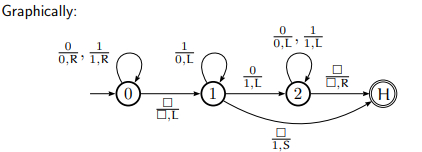# Construct a TM for adding 1 to a binary natural number?

A Turing machine (TM) can be formally described as seven tuples −

(Q,X, ∑, δ,q0,B,F)

Where,

• Q is a finite set of states.

• X is the tape alphabet.

• ∑ is the input alphabet.

• δ is a transition function: δ:QxX->QxXx{left shift, right shift}.

• q0 is the initial state.

• B is the blank symbol.

• F is the final state.

Binary numbers

1 = 1

2 = 10

3 = 11

4 = 100

5 = 101

6 = 110

. . .

Algorithm

Step 1 − Move to the right end of the string.

Step 2 − Repeat:

If the current cell contains 1, write 0 and move left until the current cell contains 0 or blank.

Step 3 − Write a 1.

Step 4 − Move to the left end of the string and halt.Let ❑ call it as B.

The TM instructions are as follows −

(0, 0, 0, R, 0) Scan right.

(0, 1, 1, R, 0) Scan right.

(0,B ,B , L, 1) Found right end of string.

(1, 1, 0, L, 1) Write 0 and move left with carry bit.

(1, 0, 1, L, 2) Write 1, adding done.

(1,B , 1, S, Halt) Write 1, done and in proper position

(2, 0, 0, L, 2) Scan left.

(2, 1, 1, L, 2) Scan left.

(2, B, B , R, Halt) Reached left end of string and halt.

Instantaneous description: 11 + 1 =2???

State 0 − B 1 1 B       Scan right

State 0 − B 1 1 B

State 0 − B 1 1 B       Finished scan.

State 1 − B 1 1 B       1 to 0

State 1 − B 1 0 B       1 to 0

State 1 − B 0 0 B       0 to 1

Halt − B 1 0 0 B       Done!